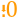# Round Down

 Block Group: Number Formatting Icon:The Round Down block rounds a number down, toward zero if positive and away from zero if negative.

For information on using dataflow blocks, see Dataflow.

For accepted and excluded arguments of Number Formatting blocks, see Number Formatting.

## Input/Output Properties

The following properties of the Round Down block can take input and give output.

• input (number)
• precision (number)

input specifies any real number that you want rounded down.

precision specifies the number of digits after the decimal to round the number to. A value of 0, less than 0, or null rounds the number down to an integer.

## Output Property

The following properties of the Round Down block can give output but cannot take input.

• output (number)

output returns the rounded-down number.

## Example

The following image shows an example of the Round Down block. In this example, the number 76.9 is rounded down to an integer.